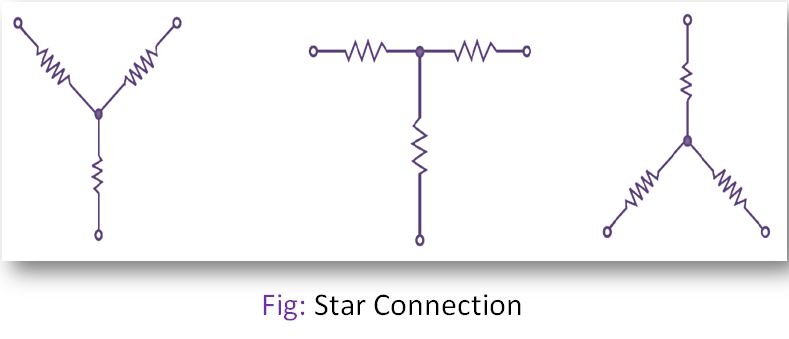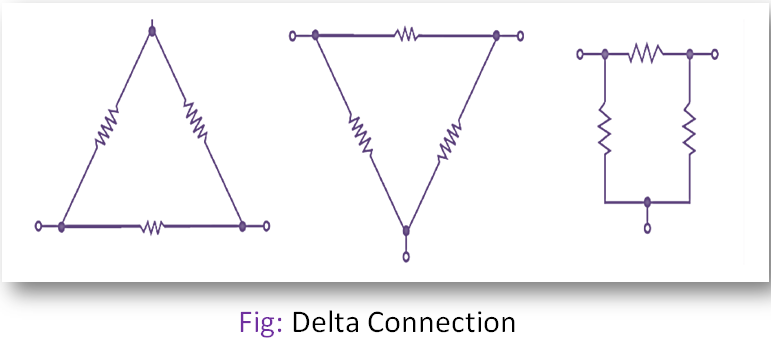# Star Delta Transformation Solved Problems

## Star Connection:

When three resistance is so connected that either the starting point or the finishing point of each resistance is shorted together, then it is called a star connection.## Delta Connection:

When three resistances are so connected that the finishing point of one resistance is connected to the starting point of the other resistance, then is said to be a delta connection.When it is found to be very difficult to solve the problems with the help of Kirchhoff’s law, then these complicated electrical networks can be solved easily by replacing successively star connections with equivalent delta connections and delta connections with equivalent star connections.

## Star to Delta Transformation:

The equivalent delta resistance can be found out is this way. Star to Delta Transformation is the summation of two-star resistances, where the delta resistance meets plus the product of these two-star resistances divided by the third-star resistance.

## Delta to Star Transformation:

The equivalent star resistances can be found out in this way. Delta to Star Transformation is the product of the two delta resistance, where the star resistance meets its end divided by the summation of three delta resistances.